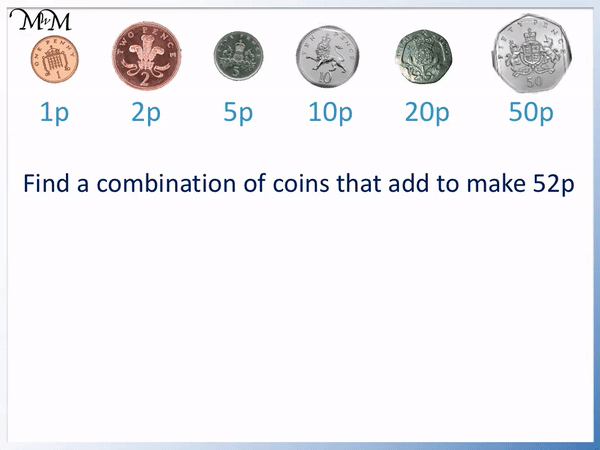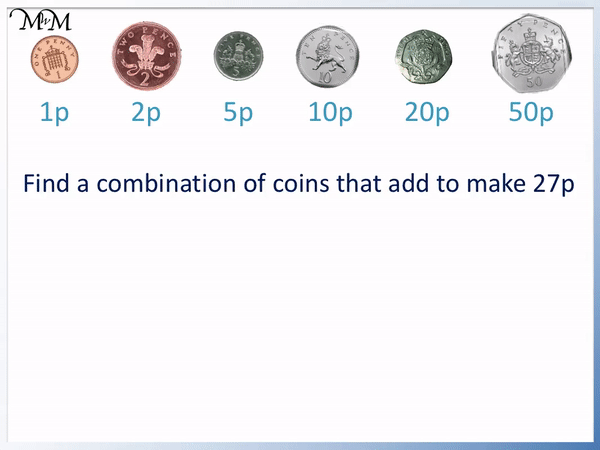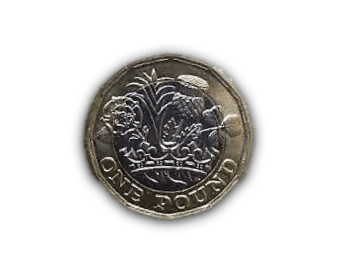# Combining Coins to Make an Amount

Combining Coins to Make an Amount• We will combine a set of coins to make a value of 36 pence.
• 50 pence is too large so we can cross it out.
• We can take one 20 pence.
• We cannot add another 20 pence because we would have more than 36 pence.
• We cross out 20 pence.
• We can add ten pence more to make 30 pence.
• We cannot add another ten pence and so, we cross it out.
• We can add 5 pence to make 35 pence.
• Finally, 1 pence more makes 36 pence
• We can make 36 pence from a 20p, 10p, 5 and 1p coin.

Cross the coins off if we cannot add another without making a value larger than we need.Supporting Lessons# How to Add or Combine Coins to Make a Particular Value

To add coins to make a particular value, start by looking at the largest value coins. If the coin’s value is larger than the value being made, cross off the coin. Continue to add coins, crossing them off if adding them to the total takes the total over the value needed.

Here is an example of making a particular value.

Find a combination of coins that add to make 52 pence.

50p is less than 52p and so, we can take one 50p coin.

If we add another 50p coin, we have too much. We need 2 more pence to get to 52p.We can make 52p using a 50 pence coin plus a 2 pence coin.

In this example we will make 61 pence.If we add another 50p, we will have more than 61p and so, we cross it off.

If we add a 20 pence to the 50p coin, we have 70p, which is more than 61 pence. We do not take a 20 pence coin.

If we add a 10 pence coin to the 50p coin, we have 60p.

We need 1 more pence to make 61p.

61 pence can be made from a 50 pence coin, a 10 pence coin plus a 1 pence coin.

In this example, we will look at how to make a value of 27 pence using coins.

50 pence is the largest pence coin and it is too much. It is larger than 27 pence. We cross it off immediately.

We can take one 20 pence coin.If we add a 10 pence coin to the 20 pence coin, we have 30 pence. This is too much and so, we cross off the 10 pence coin.

We can take one 5 pence coin.

20 + 5 = 25. We have 25 pence so far.

We can take a 2 pence coin to make 27 pence.

In this example we will make 36 pence.

50 pence is already too large and so, we cross it off.We can take a 20 pence.

Another 20 pence would make 40 pence and this is too large. We cross off the 20 pence coin now.

We can instead add a 10 pence to the 20 pence coin. This makes 30 pence.

Adding another 10 pence to 30 pence would make 40 pence and so, we do not add another 10p coin.

We can add a 5p coin to make 35 pence.

We can finally add a 1 pence coin to make 36 pence.

In this example we are finding a group of coins that add to make 44 pence.

Again, 50p is too large.We can take a 20 pence coin.

This time we can add a second 20 pence coin to make 40 pence.

We cannot take a third 20p coin because 40 pence plus 20 pence equals 60 pence. We cross off the 20 pence coin.

We continue to go through the coins to see if we can add to the 40 pence we have so far.

Adding 10 pence takes us to 50p, which is too large.

Adding 5 pence takes us to 45p, which is too large.

We need 4 more pence to get from 40p to 44p.

We can add two 2 pence coins.Now try our lesson on British Money Maths: Introducing Pound Sterling Coins where we learn about pound sterling coins.error: Content is protected !!